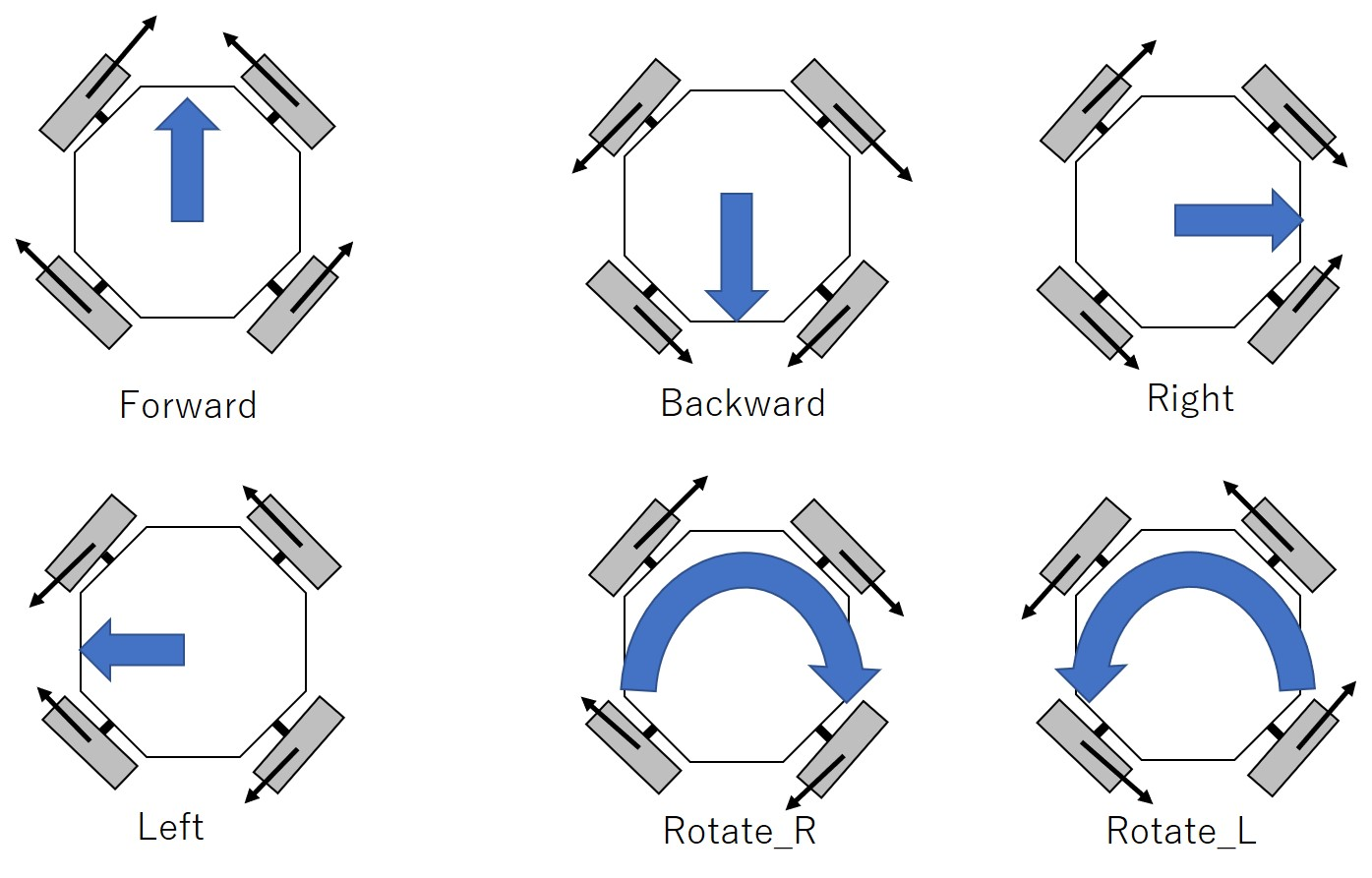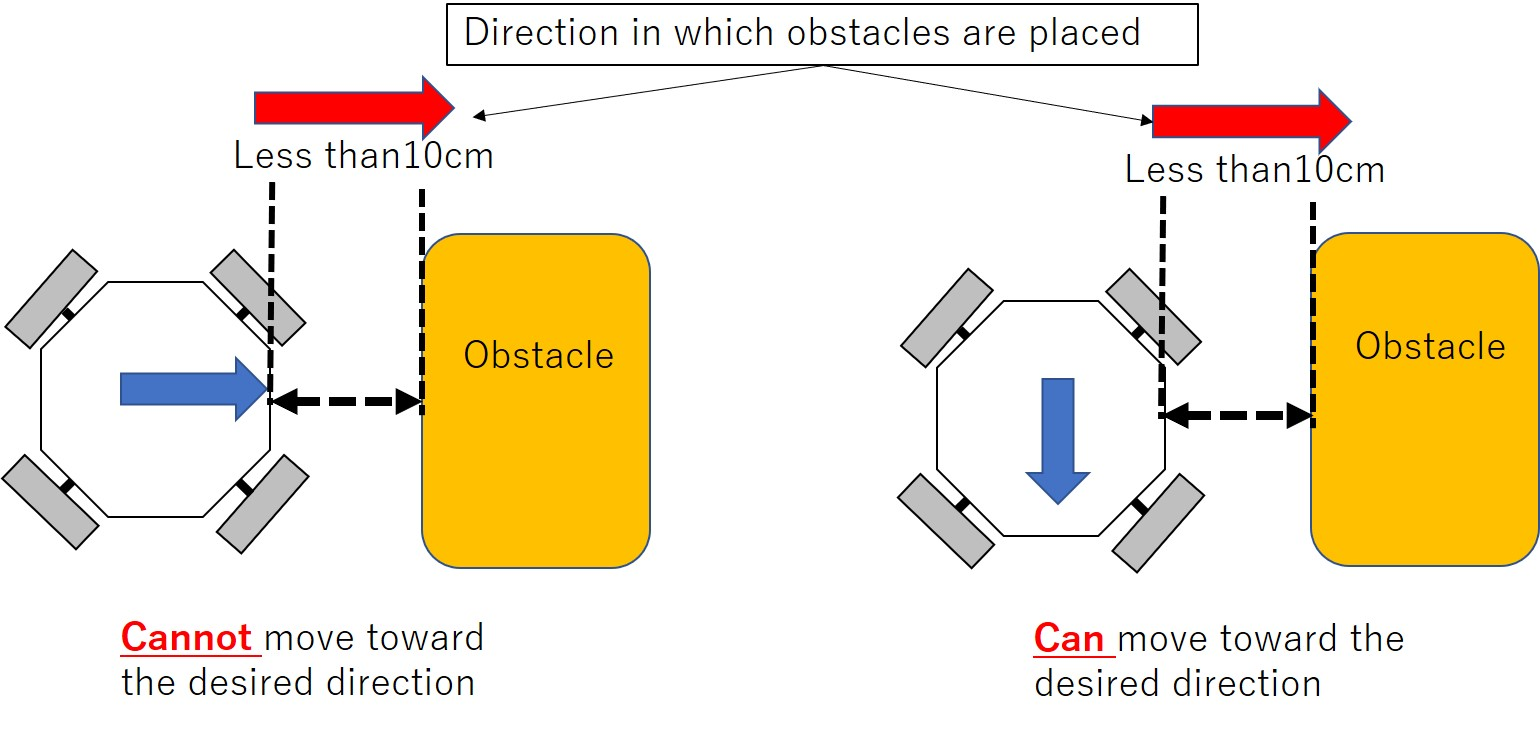# Embedded Programming¶

In this page, I describe coding works for my project. In my project, I wrote C language for Attiny44, Arduino code for Satshakit, and Java forr smartphone application..

## C language¶

C language for Attiny44 was for HC-SR04. This code was used to detect obstacles in front of the robot and send a 1-bit digital signal to Satshakit to let the Satshakit know there is an obstacle. The detailed information including code is described the assignment week 11.

## Arduino IDE¶

The code edited in Arduino IDE is for mainly controlling four Omni-directional wheels based on the information from HC-SR04 (distance sensor) and HC05(Bluetooth).

When the robot receives characters defining the required directions, the robot runs toward the desired directions. Available directions are forward, backward, left, right, rotating right direction, and rotating left direction. To achieve these movements, rotating patterns for each wheel are defined as shown in the below figure.As described week11, when there is an obstacle within 10 cm in front of the robot, the robot should stop moving to avoid collapsing the obstacle. I coded when one certain HC-SR04 detects an obstacle, the robot cannot run toward only that direction (can run toward other directions). The below code(part of loop function) enables the robot to achieve the function.

```void loop() {
char c;
int input;
Serial.print(D_front);
Serial.print(D_left);
Serial.print(D_right);
Serial.println(D_back);

while (serialBT.available()){
if(D_front==0 || D_back==0 || D_right==0 || D_left==0){
c = (char)serialBT.read(); //get each char and fill string
if(c=='a' && D_front==0){//forward
Serial.print("forward");
Serial.print("\n");
Direction=1;
Forward();
delay(100);
}
else if(c=='b' && D_back==0){//back
Serial.print("back");
Serial.print("\n");
Direction=2;
Backward();
delay(100);
}
else if(c=='c' && D_left==0){//left
Serial.print("left");
Serial.print("\n");
Direction=3;
Left();
delay(100);
}
else if(c=='d' && D_right==0){//right
Serial.print("right");
Serial.print("\n");
Direction=4;
Right();
delay(100);
}
else if(c=='e'){//rotate_right
Serial.print("RotateR");
Serial.print("\n");
Rotate_R();
delay(100);
}
else if(c=='f'){//rotate_left
Serial.print("RotateL");
Serial.print("\n");
Rotate_L();
Stop();
delay(100);
}
delay(100);
}
}

if (Direction==1 && D_front==1){Serial.println("ObstacleFront");Stop();}
else if(Direction==2 && D_back==1){Serial.println("ObstacleBack");Stop();}
else if(Direction==3 && D_left==1){Serial.println("ObstacleLeft");Stop();}
else if(Direction==4 && D_right==1){Serial.println("ObstacleRight");Stop();}

delay(10);
}
```

The variables “Direction” means that the robot recognizing it is running a certain direction (for example, Direction=1 means the robot is running FORWARD direction). In my plan, only when directions of moving and in which obstacles are placed are the same, the robot should stop moving. Then, I defined this information.## Smartphone application using Bluetooth¶

As I described week16, a smartphone is a user interface to control the robot through Bluetooth communication. By using the application, the user can send commands of directions toward which the robot moves.

*Arduino code
C language code can be donwload from week11.